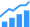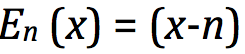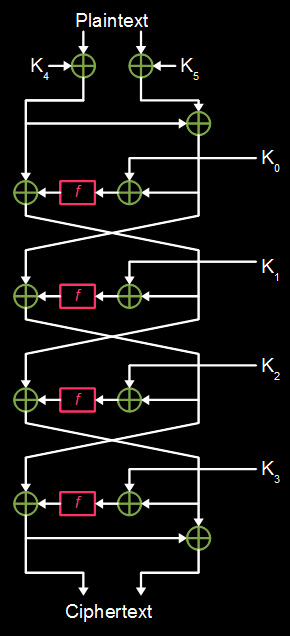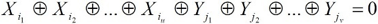# The Secret World Of Ciphers

What’s changing in this clandestine sector, and what does it really take to be an expert?The arena of creating secure environments in the hardware and software industries is somewhat shrouded in mystery and misunderstanding. Certainly, some types of ciphers are relatively straightforward and uncomplicated. For example, there is one called the Caesar cipher, which is one of the most prolific, and simple encryption techniques.

Basically, this is simply aligning two alphabets and shifting one of them by some number of positions. Mathematically this can be represented by:; on the sending side, and, on the receiving side. Figure 1 shows a simple cipher wheel, and an example of the shift. All one needs to do is align the wheels correctly and substitute the characters.

The most basic configuration simply shifts one letter. In that case, an A in one alphabet is simply a B in the other. That shifts everything one letter to the right, or left, depending on whether one is the sender, or recipient. This has been a staple of high school encryption between students for years.

Another simple scheme, termed Atbash, can be traced back to the original Hebrew alphabet. It is transposing the letters of the alphabet in reverse. For example, A becomes Z, B becomes Y, and so on.

On the other end, there are super complex ciphers, some so secret that no one really knows about them except the originators. But there is not likely a single candidate that can be considered the “top” cipher. At this scale, it really depends upon the application because there are several elements that can be combined to provide different functions of the cipher. But generally, complex ciphers involving AES, RSA, or stream and block can be made very strong and secure. Then, of course, there is QKD, the granddaddy of all unbreakable ciphers.

Below the surface of all of this is a lot of complexity, both in what is going on and who is doing it. Let’s drill down on this a bit.

Who are the cipher experts?
“Most people shouldn’t try to develop ciphers,” observes Richard Newell, senior principal product architect for the SoC Products Group at Microsemi. “It is very difficult to get a cipher correct, and one really needs to be an expert. And there are not that many experts in the world.”

Steven Woo, Vice President of Enterprise Solutions Technology at Rambus agrees: “One of the key challenges is how to develop a cipher that continues to be difficult to crack against the increasing capabilities of computing power, over time.” This is a very important metric in cipher development.

There is a lot more to developing ciphers than just writing some code to encrypt data. “The danger here is that when you first learn about developing ciphers, there is a tendency to think that it is not all that difficult,” says Newell. “That can lull you into thinking you know more than you do.”

In most of these cases, the end result is failure of the cryptographic system. There are other metrics that developers also need to consider as well.

“Given enough time and resources, any cipher can be cracked,” says Woo. “One of the most important aspects of being a cipher developer is knowing how to find the sweet spot where it becomes too much effort to crack, and it approaches the point where it isn’t worth the hackers’ time and money to crack them and they give up. It also is important to understand how the keys are constructed, so you can comprehend the time and effort it takes someone to crack the cipher.”

From a design perspective, there is a very deep well of knowledge required to design optimal ciphers. To become a top-notch cipher designer, one has to first become an expert in cryptanalysis. Cryptanalysis is the study of the science and methodologies of analyzing cryptographic systems, understanding how they work, and knowing how they can be broken. This requires knowledge of how to analyze cryptographic algorithms, understanding peripheral areas such as how side channel attacks work. It also requires being able to discover weaknesses in algorithmic implementation, not just the algorithm itself.

It is very difficult to know what types of mathematical attacks are possible on a cipher, which is a prerequisite to designing a good cipher. It takes a long time and a lot of knowledge just to begin to understand what is going on from a mathematical perspective. Add to that the metrics’ practicality, and it takes a lot of work and comprehension to become a top cryptanalysis.

Additionally, one has to be an expert in cryptology, which is the overall science, and cryptography, which is generally seen as protecting the attack objective (data) using ciphers.

What’s new
Two areas that are hot topics in today’s cryptography are linear and differential cryptanalysis. These are the ability to measure any type or mathematical imperfection within the cipher than can be exploited by an adversary.

“Traditional thinking, with current encryption methods, is that the algorithm doesn’t need to be secret,” says Woo. “It is the key that needs to be secret.”

That may have been the mindset so far, but with today’s enormous processing capabilities, keeping the key secret may not be enough. “By monitoring the noise coming off the chip, one is able to infer something about the secret key because you know the algorithm,” Woo says.

That’s one of the reasons both differential and linear cryptanalysis work, and why there is new momentum to protect the algorithms as well as the keys. The complexity of multi-round ciphers, such as DES, make them very difficult to crack, even if someone had acquired certain corresponding plaintext and ciphertext. It is still it still very difficult to determine the corresponding key that has been used.

Differential cryptanalysis
That’s where differential cryptanalysis comes into play. Let’s say, through some unusual circumstance, a large quantity of corresponding plaintext and ciphertext blocks has been acquired that relates to a particular unknown key. Using differential cryptanalysis, the analyst (or hacker) often can obtain clues about some of the bits of the unknown key. That considerably shortens the time it takes to obtain the key.Fig. 2: Differential cryptanalysis process. Source: theamzingking.com

For example, if two rounds of DES has been run and now the input and output is known, it becomes relatively easy to determine the two sub-keys used because the outputs of both f functions have been realized. The approach is relatively straightforward.

The technique is to us an S-box. Each S-box, has four possible inputs that will produce the known output. In this example, each sub-key is 48 bits long. However, the key is, itself, is 56 bits long. Now it becomes and exercise in problem solving. The sub-key is divided into eight groups of six bits, and it becomes a matter of determining which of the four possibilities is true for each group of six bits.

This works well for a two-round DES. If the number of rounds increases to four, it increases the difficulty by at least an order of magnitude. However, there is a truism that no matter how many round are run, the output still depends on the input and the key. Assuming a limited number of rounds and no flaws in the S-boxes, eventually there will be some successes where one or more bits of the output will have a certain measure of correlation to a combination of input bits and key bits.

Under ideal circumstances, that correlation should be absolute with respect to the key bits. That’s because in this example there is only one key to solve. That doesn’t mean it will work every time, or even most of the time. There are still a lot of pairs to test, with respect to the input and output bits.

Now, extrapolate this with increasing rounds of DES. Obviously, there is a nonlinear relationship in the increase in input and output variables, so the complexity increase non-linearly, as well.

In this case, differential cryptanalysis can take a different approach, namely to find subtler correlations. For example, rather that assuming that a specific bit is 1 at the input — and then it will be a 0 or 1 at the output, based on the above methodology — the expectation is that changing the bit will or will not change a bit at the output.

Differential cryptanalysis can develop a pattern of what bits change at both the input and output. This technique is all about simplification. It takes complex plaintexts or ciphertexts and looks for anomalies that behave like simpler approximations. The basic principle is that whatever cipher being analyzed has the characteristic that if there exists a constant X, such that given many pairs of plaintexts A, B, such that B = A XOR X, if a certain statement is true about the key, E(B,k) = E(A,k) XOR Y for some constant Y will be true with a probability somewhat higher than that given simply by random chance.

Simply stated, differential cryptanalysis has the ability to remove some of the minutiae by narrowing the field to more probabilistic possibilities than just brute force alone can.

Linear cryptanalysis
In contrast, the linear cryptanalysis method does not search for isolated points where a block cipher may behave as a simpler cipher. Developed by Mitsubishi’s Mitsuru Matsui [see reference 1], this approach addresses the complete cipher. Its methodology revolves around attempting to simplify the block cipher as a whole rather than its pieces by trying to develop a simpler approximation of the complete block cipher.

The principle of this can be likened to summing many single-dimensional scans to produce a two-dimensional slice through an object in computer-assisted tomography. For a great many plaintext-ciphertext pairs, this works. The first process is to find a key that would produce that pair from the simplified cipher. This produces key bits that are likely to have the value of the corresponding bit of the key for the real cipher. How it does it is like this.

As with DC, this assumes some knowledge of the set of random plaintexts and corresponding ciphertexts. The basic concept is to approximate, with a linear expression, the operation of a portion of the cipher using an exclusive-OR (XOR), symbolized by. The equation is in the form of:Where Xi represents the i^th bit of the input; X = [X1, X2, …] and Y^j represents the j^th bit of the output Y; = [Y1, Y2, …]. Essentially, this equation represents the XOR “sum” of the u input bits and the v output bits. In linear cryptanalysis, this formula can be used to find variants that likely have a probability of occurrence higher, or lower than the norm.

Why this works is rather interesting. If one just takes randomly selected values for the u + v bits and sticks them into the equation, the probability of the expression holding would be, precisely, 1/2. That would go on infinitely. However, in linear cryptanalysis the deviation from the probability of 1/2 is that an expression to hold is what can be exploited. So as the deviation moves further away from the probability of 1/2, the more identifiable the results of the application of linear cryptanalysis.

Here is an example of that. Let’s make the assumption that a given equation will yield a 1, with a probability just slightly higher than 1/2. Then, for a given key XOR-ing, running a high number of plaintexts, with corresponding ciphertexts, will yield a result that will be a 1, slightly more often that 0. That is the deviation discussed in the previous paragraph. Once a sufficient number of plaintext/ciphertext pairs is acquired for each pair, the equation is an XOR between a few bits of each of the plaintext, the ciphertext, and the key. Because for each pair the plaintext and ciphertext is known, the equation will yield the value of the XOR of the few key bits. Why this works is that the equation is “right” just a light percentage more often that it is work, so with a sufficient number of pairs, a pattern starts to emerge as the majority, which can be used with a strong probability that it is a bit of the key. If this process is repeated enough times, theoretically, all of the key bits should be able to be determined.

Bottom line
Both of these methods are effective cryptography approaches. But just skimming the surface of what’s needed to design and reverse engineer the wide range of ciphers out there shows just how challenging it is to be at the top in this field.

To be a top cryptanalyst, one has to be both a designer and an attacker. One also has to understand the advanced and complex mathematics, such as differential and linear analysis and others, to be able to build and tear down ciphers. In addition, one has to understand the myriad of ciphers currently in use, such as RSA, AES, SHA, ECC, and all of their nuances.

Most of the world has no clue to the complexity of cryptanalysis. Some think they do, which is why there are so many failures of cryptography today. But eventually those who need cryptography will turn to the real experts – especially when the world of the IoE really starts to develop.

Glossary
DES – Data Encryption Standard
DC – Differential Cryptanalysis
ECC – Eliptical Curve Cryptograhy
LC – Linear Cryptanalysis
QKD – Quantum Key Distribution
RSA- Initial letters of the surnames of Ron Rivest, Adi Shamir, and Leonard Adleman, its creators
SHA – Secure Hash Algorithm
XOR – A digital logic gate with two or more inputs, and one output that performs exclusive disjunction. The output of an XOR gate is true only when exactly one of its inputs is true, rahter than either input

Reference 1: M. Matsui, “Linear Cryptanalysis Method for DES Cipher”, Advances in Cryptology, EUROCRYPT ’93 (Lecture Notes in Computer Science no. 765), Springer-Verlag, pp. 386-397, 1994.programify says: